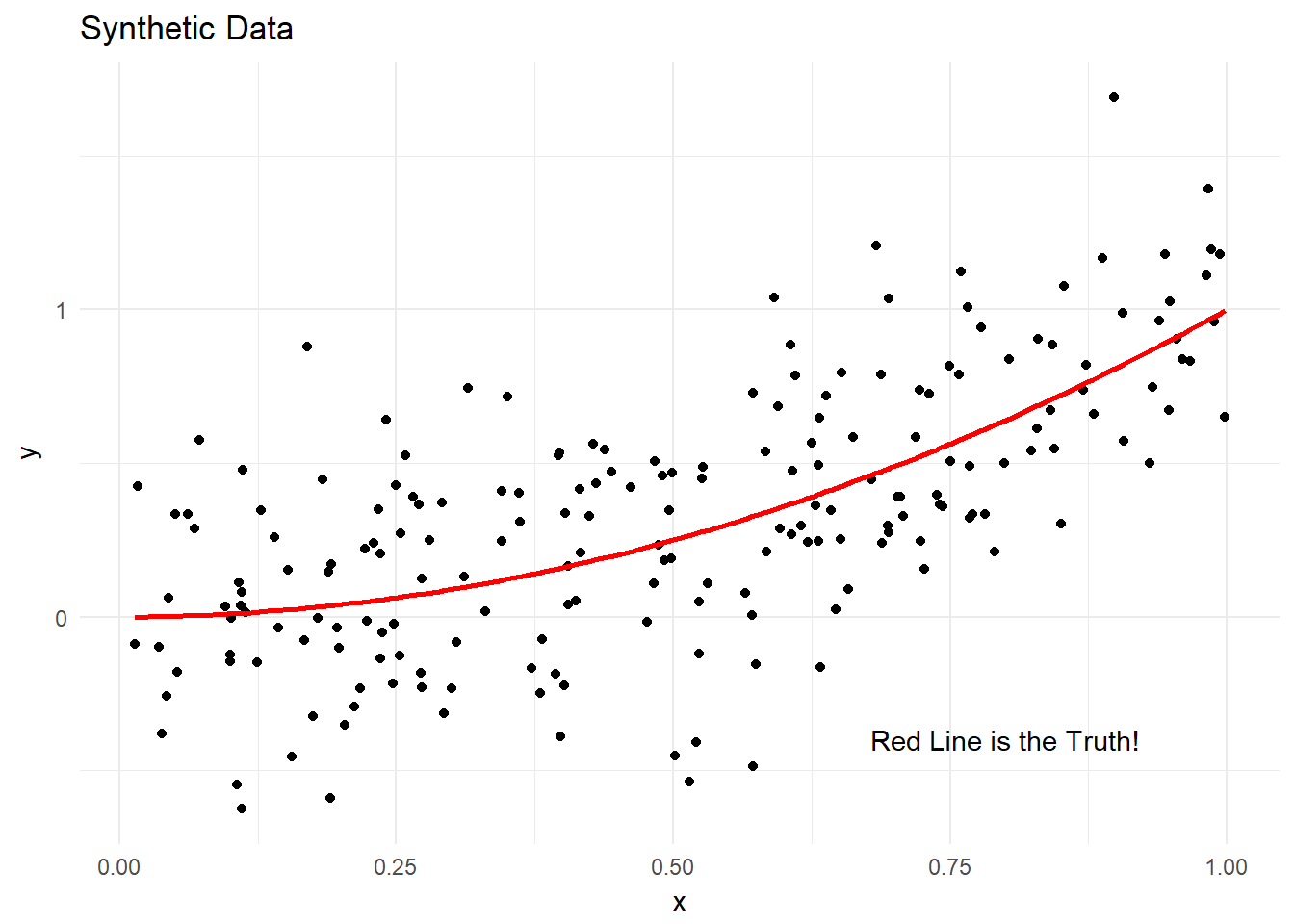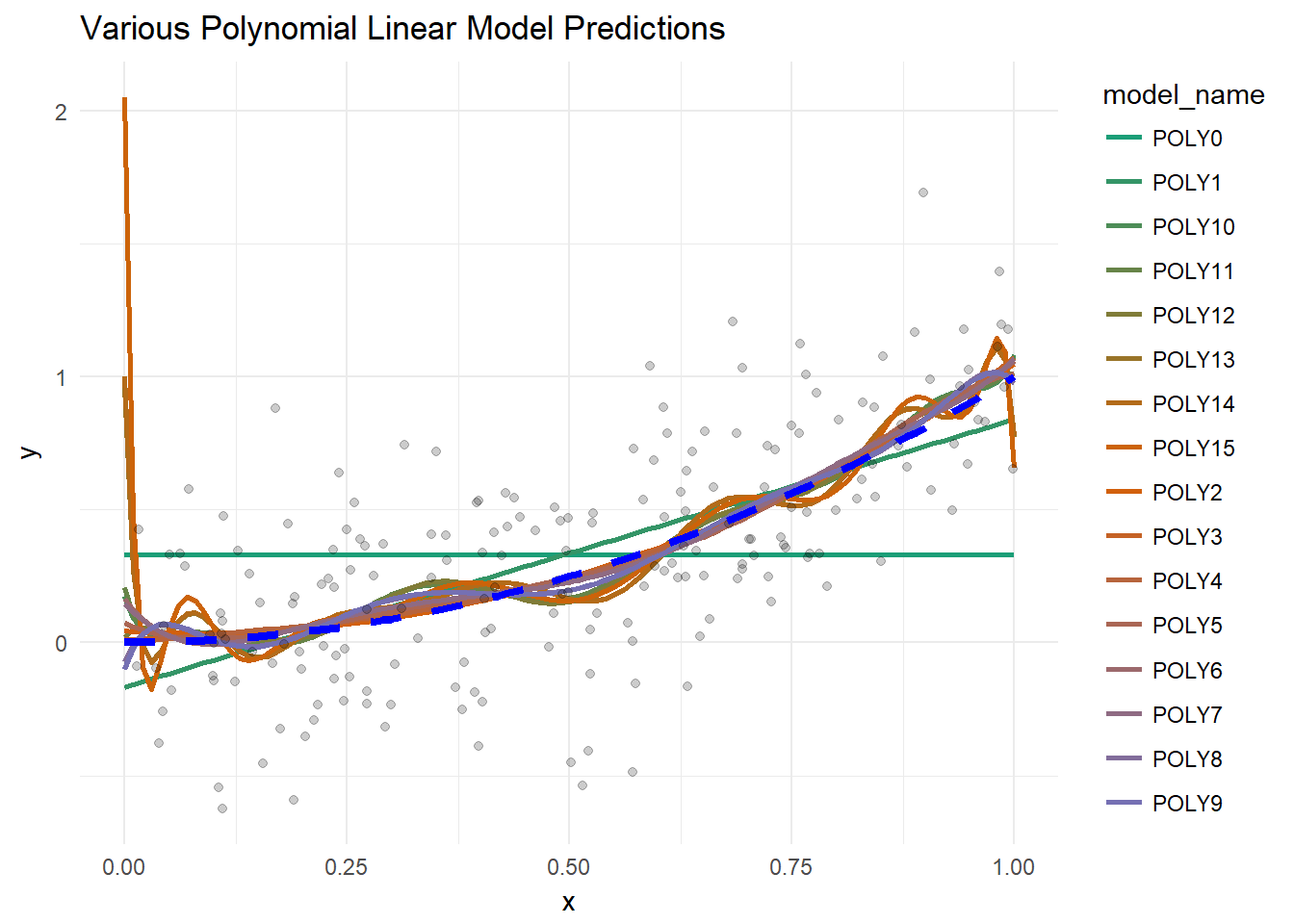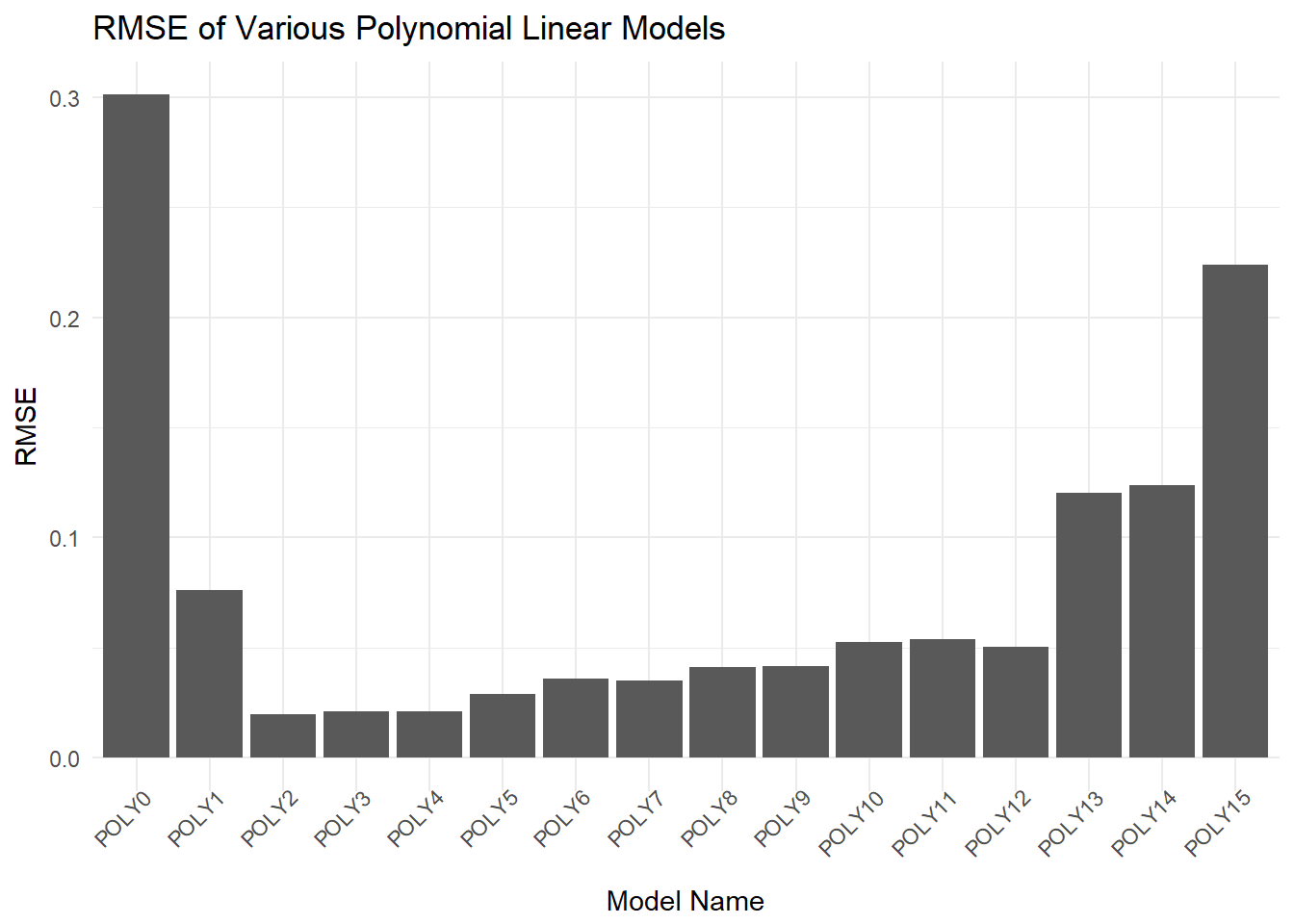# Glossary

Below I have a selection of techniques I use with purrr in this document, for your convenience. Note that map always returns a list.

``````purrr::map(data, function) # use function on each element of data, return list
purrr::map(data1, data2, function) # use function with both sets of data

# Create a new variable called foo which is the result of using my_function on each element of x in the data frame.
df %>% mutate(foo = map(x, my_function))

# Extract stuff from bar into new column foo, by way of an anonymous function.
# In essence, baz is an element of bar and stuff is extracted from it.
df %>% mutate(foo = map(bar, function(baz) baz\$stuff))``````

## Introduction

I wanted to look at the infamous bias-variance tradeoff in an empirical way by using different model parameters and seeing how they affected the error.

I also figured this might be a nice way to clearly demonstrate the use of list columns in data frames and nested data frames for times when you have multiple models to compare. The idea is that you can’t put data frames as columns in other data frames, but you CAN put lists as columns in a data frame. And guess what lists can hold? Data frames and any other object you so desire.

As a result we can use functional programming ideas to perform tasks easily on each of these elements of these list columns, ensuring we consistently perform our manipulations and calculations on each element. These ideas are easily accessible by using `purrr` from the tidyverse.

``````library(tidyverse)
library(caret)
library(RColorBrewer)``````

Functions:

``````truth <- function(x) x^2

sim_sample <- function(fun, num = sim_size) {
sim <- tibble(x = runif(num))
sim\$y <- (fun(sim\$x) + rnorm(num, sd = 0.3))
sim
}

safe_lm <- function(model_data, model_degree) {
# Prevents a 0 model degree from causing an error and returns a model of
# degree 0. Model data must have x and y columns
if(model_degree == 0){
lm(y ~ 1, data = model_data)
} else {
lm(y ~ poly(x, degree = model_degree), data = model_data)
}
}

get_pal <- function(pal_name, number) {
colorRampPalette(RColorBrewer::brewer.pal(3, pal_name))(number)
}``````

## Synthetic Data

Let’s apply a `lm` to a set of simulated data, in this case y = x².

First off we have to generate the synthetic data with a random error around the “truth”. I have plotted this data below with the actual underlying function as a line.

``````sim_size <- 200

synth_sample <- sim_sample(truth, sim_size)
actual <- tibble(synth_sample\$x, truth(synth_sample\$x))

synth_sample %>%
ggplot(aes(x, y)) + geom_point() + labs(title = "Synthetic Data") +
geom_line(aes(x, truth(x), group = 1), colour = "red", size = 1) +
theme_minimal() +
annotate("text", x = 0.8, y = -0.4, label = "Red Line is the Truth!")``````Next we need to create a tibble of models to allow use of `purrr::map` tools on the different models. Note I had to create a “safe” version of lm that accepted `degree = 0` when using a polynomial formula.

This is the first use of the `purrr::map` function, it is used here to generate the name of each model by pasting “POLY” to each member of the sequence 0:10.

Note that I also put the same modelling data into each row of the data frame, this will be important in the next step

``````generate_modelling_df <- function(max_poly_degree, model_data) {
tibble(
model_name = map_chr(0:max_poly_degree, function(x) paste0("POLY", x)),
poly_num = 0:max_poly_degree,
synth_data = list(model_data)
)
}

models_df <- generate_modelling_df(15, synth_sample)

glimpse(models_df)``````
``````## Observations: 16
## Variables: 3
## \$ model_name <chr> "POLY0", "POLY1", "POLY2", "POLY3", "POLY4", "POLY5...
## \$ poly_num   <int> 0, 1, 2, 3, 4, 5, 6, 7, 8, 9, 10, 11, 12, 13, 14, 15
## \$ synth_data <list> [<# A tibble: 200 x 2,            x           y,  ...``````

## Many Models

From there it is trivial to use a map to generate the models since we already have the modelling data (repeated in each row) and the input parameter for the polynomial linear model.

``````models_df <-
models_df %>%
mutate(model = map2(synth_data, poly_num, safe_lm))

glimpse(models_df)``````
``````## Observations: 16
## Variables: 4
## \$ model_name <chr> "POLY0", "POLY1", "POLY2", "POLY3", "POLY4", "POLY5...
## \$ poly_num   <int> 0, 1, 2, 3, 4, 5, 6, 7, 8, 9, 10, 11, 12, 13, 14, 15
## \$ synth_data <list> [<# A tibble: 200 x 2,            x           y,  ...
## \$ model      <list> [<0.3299799, 0.142938459, -0.281888684, 0.25389048...``````

Similarly for the predictions, but first we generate a “grid” in order to make predictions at regular intervals of x. Think of this like our test data in a normal analysis. Note that I also generated a nested data frame from the raw predictions and added grid to it, so each set of predictions is available as a data frame `pred_df` with x ~ y, and also as simply a vector `pred` of raw predictions.

``````models_df <- models_df %>% mutate(grid = list(data.frame(x = seq(0, 1, 0.01))))

models_df <-
models_df %>% mutate(pred = map2(model, grid, stats::predict)) %>%
mutate(pred_df = map(pred, function(x) data.frame(y = x))) %>%
mutate(pred_df = map2(grid, pred, cbind))

models_df %>%  glimpse()``````
``````## Observations: 16
## Variables: 7
## \$ model_name <chr> "POLY0", "POLY1", "POLY2", "POLY3", "POLY4", "POLY5...
## \$ poly_num   <int> 0, 1, 2, 3, 4, 5, 6, 7, 8, 9, 10, 11, 12, 13, 14, 15
## \$ synth_data <list> [<# A tibble: 200 x 2,            x           y,  ...
## \$ model      <list> [<0.3299799, 0.142938459, -0.281888684, 0.25389048...
## \$ grid       <list> [c("0.00", "0.01", "0.02", "0.03", "0.04", "0.05",...
## \$ pred       <list> [<0.3299799, 0.3299799, 0.3299799, 0.3299799, 0.32...
## \$ pred_df    <list> [<c("0.00", "0.01", "0.02", "0.03", "0.04", "0.05"...``````

## Results

Plotting the predictions of the 11 different model show that the low and high degree polynomial models perform poorly, the former are high bias models and the latter are high variance models. Some of the high degree models will swing wildly around the actual underlying ideal (blue, dashed line).

The `purrr::unnest` step here extracts the nested data frames of predictions for each of the models. It’s a bit contrived to nest and unnest them so close to each other but I hope it gets the point across.

``````models_df %>%
unnest(pred_df) %>% # extracts the nested predictions for each model
rename(y = `.y[[i]]`) %>%
ggplot(aes(x, y, group = model_name, colour = model_name)) +
geom_line(size = 1) +
theme_minimal() +
# palette was too small, had to generate new one
scale_color_manual(values = get_pal("Dark2", nrow(models_df))) +
# original training data
geom_point(data = models_df\$synth_data[],
aes(x, y, group = 1), inherit.aes = FALSE, alpha = 0.2) +
# the underlying model y ~ x²
geom_line(aes(x = x, y = truth(x)), group = 1, colour = "blue", size = 1.5,
linetype = 2) +
labs(title = "Various Polynomial Linear Model Predictions")``````If we calculate the RMSE for each model we see the following:

``````models_df <-
models_df %>%
mutate(actual = map(grid, truth),
rmse = map2_dbl(pred, actual, caret::RMSE))

models_df %>%
# Sorts model name by polynomial degree
mutate(model_name = factor(model_name,
levels = (models_df %>%
arrange(poly_num))\$model_name)) %>%
ggplot(aes(model_name, rmse)) +
geom_bar(stat = "identity") +
theme_minimal() +
theme(axis.text.x = element_text(angle = 45, hjust = 1, vjust = 1.3)) +
labs(title = "RMSE of Various Polynomial Linear Models",
y = "RMSE", x = "Model Name")``````The error is lowest at polynomial degree 2 (which is the underlying function!) and higher elsewhere. Again we used `purrr::map` to calculate the RMSE for each model using data we had stored in each row the data frame.

## Conclusion

Hopefully you’ve picked up the following from the this little analysis:

1. Variance and bias errors are traded off against each other as you make your model more or less complex.
2. Using a data frame and `purrr` to generate and extract new columns based on other columns can be done easily and can make code easy to read. In particular, this pattern is very useful
``    df %>% mutate(foo = map(x, my_function)) ``
1. Combining purrr::map and data frames can be really handy when using multiple different models or repeating an analysis on a dataset. As an example, excluding functions, I have 4 objects and variables in my R environment while doing this analysis. Without this approach it would be very easy to clutter the environment with many, many more objects.
2. The downside is that your main data.frame can become quite large and RStudio can have a hard time rendering it. Care must be taken to not bloat the data frame unnecessarily.

A work by Cormac Nolan

cormac.nolan85@gmail.com